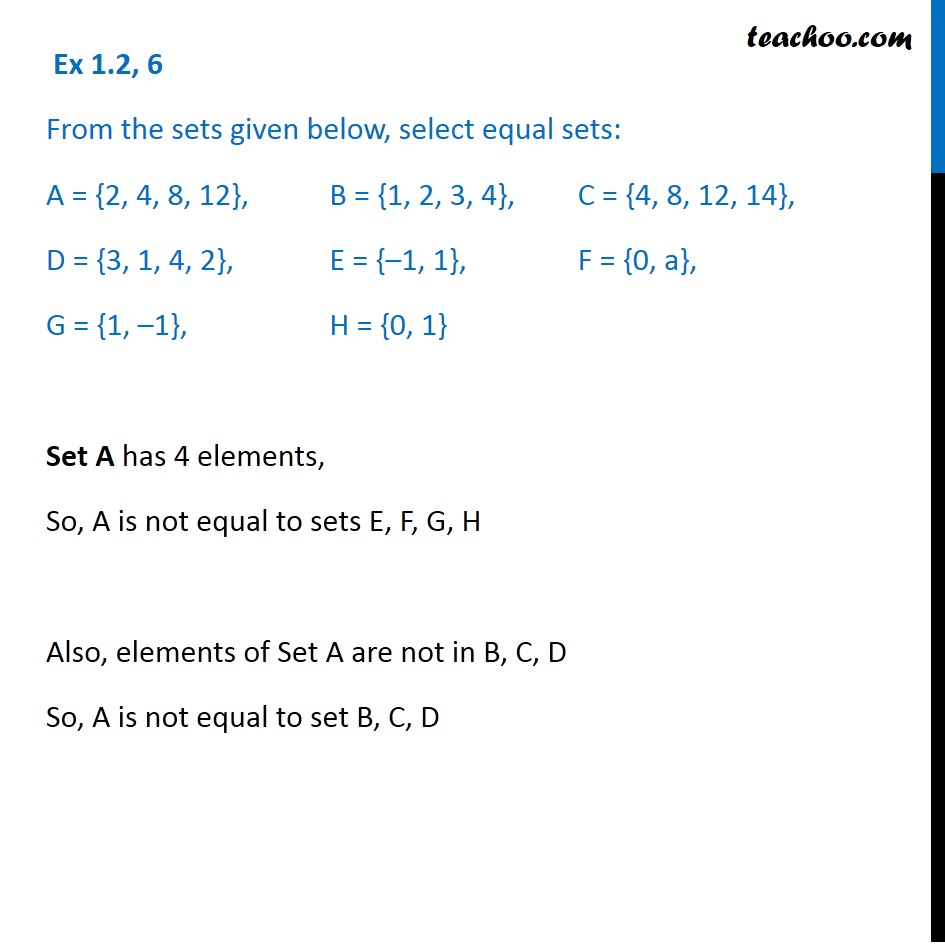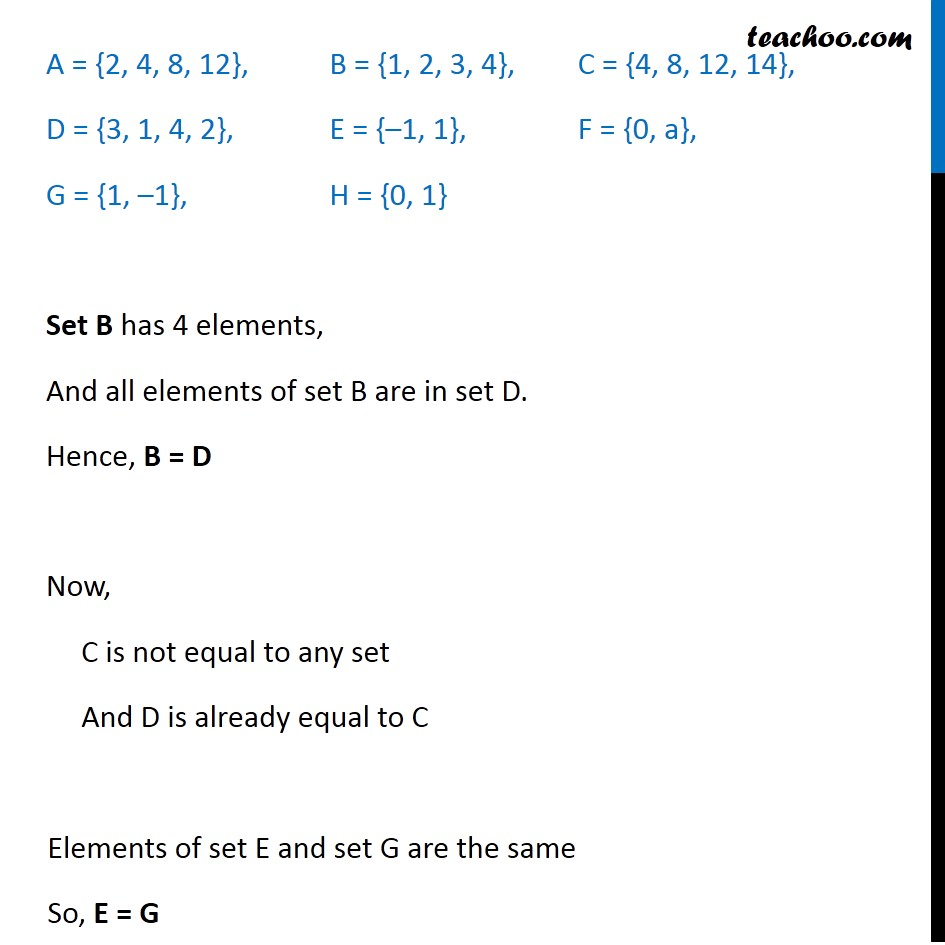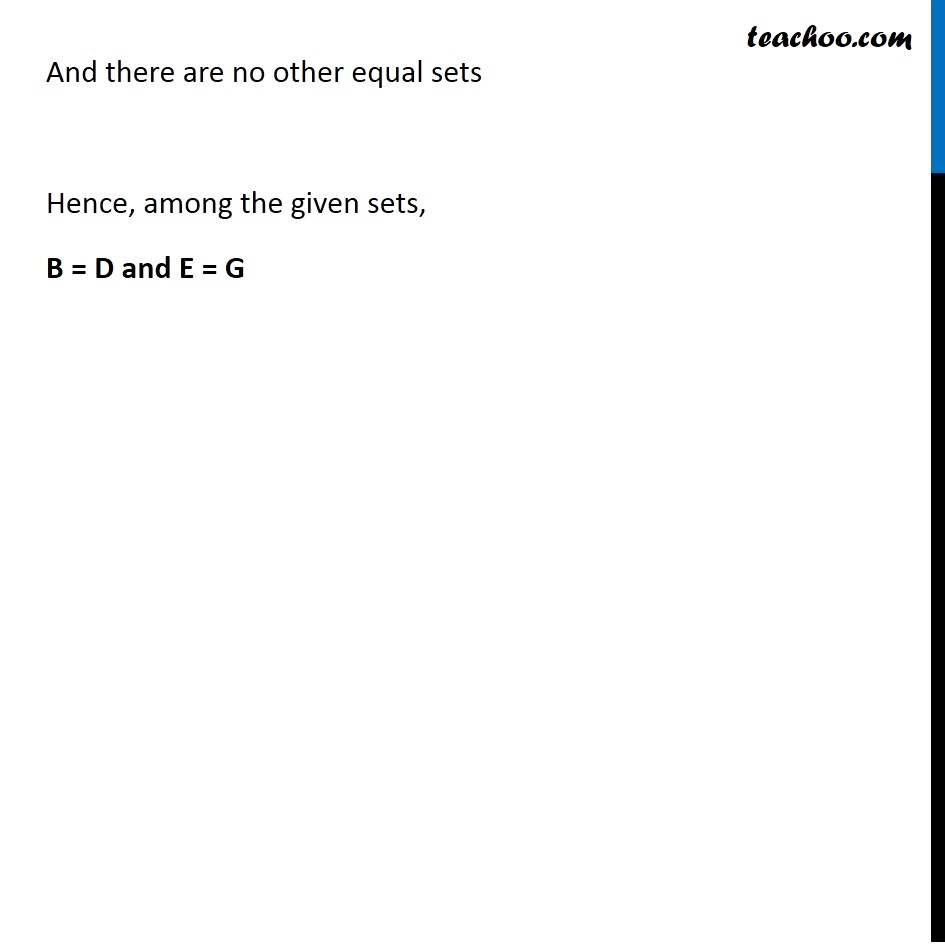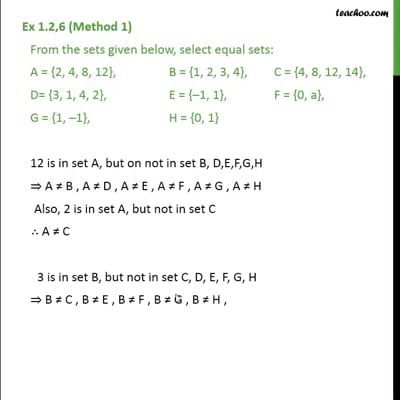Ex 1.2

Chapter 1 Class 11 Sets
Serial order wiseThis video is only available for Teachoo black users

Solve all your doubts with Teachoo Black (new monthly pack available now!)

### Transcript

Ex 1.2, 6 From the sets given below, select equal sets: A = {2, 4, 8, 12}, B = {1, 2, 3, 4}, C = {4, 8, 12, 14}, D = {3, 1, 4, 2}, E = {–1, 1}, F = {0, a}, G = {1, –1}, H = {0, 1} Set A has 4 elements, So, A is not equal to sets E, F, G, H Also, elements of Set A are not in B, C, D So, A is not equal to set B, C, D A = {2, 4, 8, 12}, B = {1, 2, 3, 4}, C = {4, 8, 12, 14}, D = {3, 1, 4, 2}, E = {–1, 1}, F = {0, a}, G = {1, –1}, H = {0, 1} Set B has 4 elements, And all elements of set B are in set D. Hence, B = D Now, C is not equal to any set And D is already equal to C Elements of set E and set G are the same So, E = G And there are no other equal sets Hence, among the given sets, B = D and E = G